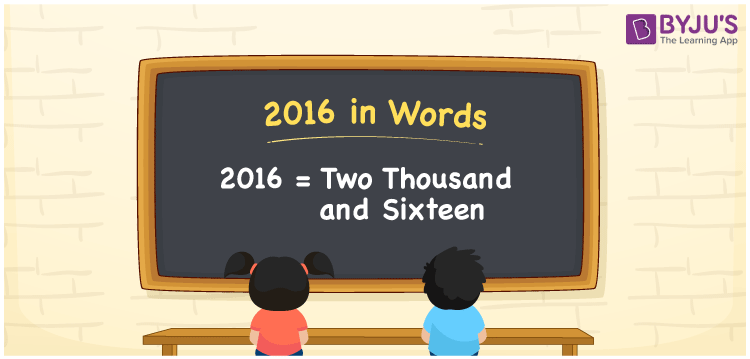# 2016 in Words

The number name of 2016 is “Two thousand sixteen. The number 2016 is a natural number that can be written using the place value of a number. For example, John made a transaction of Rs. 2016. This can be written as “John made a transaction of Rs. Two thousand sixteen”. We will discuss 2016 in words and the procedure for writing it in this article.

 2016 in Words: Two Thousand Sixteen. Two Thousand Sixteen in Numerical Form: 2016.

## 2016 in English Words## How to Write 2016 in Words?

To convert the number 2016 into words, find the place value of each digit. The table below shows the place values for the number 2016.

 Thousands Hundreds Tens Ones 2 0 1 6

The following is the expanded version of the number 2016:

= 2 × Thousand + 0 × Hundred + 1 × Ten + 6 × One

= 2 × 1000 + 1 × 10 + 6 × 1

= 2000 + 10 + 6

= 2016

= Two thousand sixteen

Hence, 2016 in words is two thousand sixteen.

2016 in words – Two thousand sixteen

Is 2016 an odd number? – No

Is 2016 an even number? – Yes

Is 2016 a perfect square number? – No

Is 2016 a perfect cube number? – No

Is 2016 a prime number? – No

Is 2016 a composite number? – Yes

## Frequently Asked Questions on 2016 in Words

Q1

### Write 2016 in words.

2016 in words is two thousand sixteen.

Q2

### Simplify 2000 + 16, and express in words.

Simplifying 2000 + 16, we get 2016. Hence, 2016 in words is two thousand sixteen.

Q3

### How to write two thousand sixteen in numbers?

Two thousand sixteen in numbers is 2016.